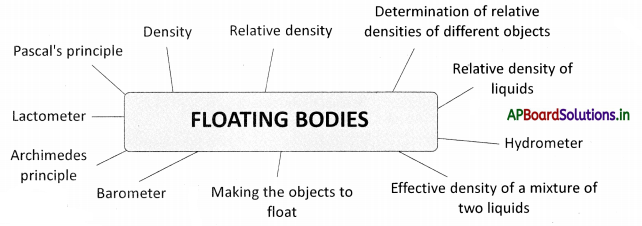# AP Board 9th Class Physical Science Notes Chapter 9 Floating Bodies

Students can go through AP State Board 9th Class Physical Science Notes Chapter 9 Floating Bodies to understand and remember the concept easily.

## AP State Board Syllabus 9th Class Physical Science Notes Chapter 9 Floating Bodies

→ Density is defined as mass per unit volume. Mass
Density = $$\frac{\text { Mass }}{\text { Volume }}$$
Unit of density = gm/cm3 or kg/m3

→ The relative density of an object is the ratio of the density of the object to the density of the water.
Relative density of an object = $$\frac{\text { Density of the object }}{\text { Density of water }}$$

→ Experimentally relative density of a solid object = $$\frac{\text { Weight of the object }}{\text { weight of water equal to the volume of the object }}$$

→ Relative density of liquid = $$\frac{\text { Weight of liquid }}{\text { Weight of the same volume of water }}$$

→ Lactometer is used to measure the purity of milk.

→ A hydrometer is used to measure the relative density of liquids.

→ Objects having a density less than the liquid in which they are immersed float on the surface of the liquid.

→ Every fluid exerts upward pressure on the objects immersed in them. If the upward pressure is less than the gravitational force on the object, the object sinks otherwise it floats on the liquid.

→ The pressure exerted by the air in our surroundings is known as atmospheric pressure. Atmospheric pressure P0 = ρhg.

→ The barometer is used to measure the atmospheric pressure at a place.→ Pressure at a depth ‘h’ in a liquid P = P0 + ρhg
P0 = Atmospheric pressure ; ρ = density of the liquid h = depth ; g = acceleration due to gravity

→ Buoyancy is the upward force exerted by the liquid on any object less dense than itself.

→ When an object is immersed in a fluid, it appears to lose weight (because of buoyancy).

→ The apparent loss of weight of an object, which is immersed in a liquid, is equal to the weight of the liquid displaced by the object. (Archimedes principle)

→ When an object floats on the surface of a liquid, it displaces a weight of liquid equal to its own weight.

→ The pressure exerted by a liquid increases with depth below the surface of the liquid.

→ Pascal’s principle states that external pressure applied to an enclosed body of fluid is transmitted equally in all directions throughout the fluid volume and the walls of the containing vessel.

→ Density: When two objects of equal volume are considered the object
with higher mass is said to be a dense object. Density is defined as mass per unit volume.
∴ Density = $$\frac{\text { Mass }}{\text { Volume }}$$
Unit of density gm/cm (or) kg/rn3.

→ Relative density: Relative density is the ratio of the density of an object to the density of water.
Relative density of an object = $$\frac{\text { Density of the object }}{\text { Density of water }}$$
(or)
Relative density of an object = $$\frac{\text { Weight of the object }}{\text { weight of water equal to the volume of the object }}$$→ Lactometer: Lactometer is a device used to measure the purity of milk. It works on the principle of relative density.

→ Hydrometer/ Densitometer: Hydrometer/Densitometer ¡s an instrument used to measure the relative density of any liquid.

→ Atmospheric pressure: Air in our surroundings exerts pressure on the surface of the earth called atmospheric pressure.
Atmospheric pressure P0 = ρhg
ρ = average density of air
h = height of the atmosphere
g acceleration due to gravity

→ Barometer: Atmospheric pressure can be measured using a barometer. The first barometer was invented by ‘Torricelli’, using mercury. The height of the mercury column at normal atmospheric pressure is 76 cm.
The increase or decrease in the height of the mercury column indicates sudden changes in the atmosphere.

→ Buoyancy: The upward force that a fluid exerts on an object less dense than itself.
(or)
Buoyancy is the ability of an object to float in a liquid.→ Archimedes principle of buoyancy: An object in a fluid is buoyant up by a force equal to the weight of the fluid the object displaces.→ Archimedes:

• Archimedes was born in 287 BC and died in 212 BC.
• He was a Greek mathematician, physicist, engineer, inventor, and astronomer.
• He is well known for his Archimedes principle.
• Among his advances in physics are the foundations of hydrostatics.